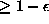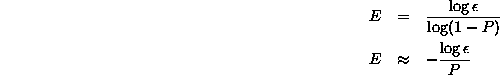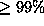Next: Uniform Random Search Up:

Why Ants are Previous: Size of Program

# Solution of the Ant Problem

Using the probability P of finding a solution we can calculate the number of program evaluations needed to ensure we find a solution (with probability). This is known as ``Effort'' required, cf. [Koza1992, page 194,]:Takingas 1% we can calculate the number of fitness evaluations E required to find at least one solution (with probability).

William B Langdon
Tue Feb 3 19:53:29 GMT 1998